# Three Dimensional Flow of Nanofluids over an Exponential Horizontal Nonlinear Sheet Saturated in Porous Medium

Three Dimensional Flow of Nanofluids over an Exponential Horizontal Nonlinear Sheet Saturated in Porous Medium

Department of Mathematics, COMSATS University of Islamabad, Lahore Campus 54000, Pakistan

A Department of Mathematics, University of Management and Technology, Lahore 54770, Pakistan

Corresponding Author Email:
Page:
555-561
|
DOI:
https://doi.org/10.18280/ijht.370224
12 December 2018
|
Accepted:
28 April 2019
|
Published:
30 June 2019
| Citation

OPEN ACCESS

Abstract:

This study analyzes the flow and heat transfer for three-dimensional stagnation point flow of water based nanofluid over an exponentially stretching surface. The base fluid for the under-discussion problem is taken to be water (H2O) and three distinct nanoparticles, namely, copper (Cu), alumina (Al2O3), and titania (TiO2). The simulations in this study assume that the surface temperature is also distributed exponentially and reduce the governing equations to a set of ordinary differential equations using a similarity transformation. Series solutions are constructed for the velocity components and temperature. Results are discussed by plotting graphs. In order to find series solution of ordinary differential equations, we employed strong analytical technique referred as optimal homotopy method (OHAM). It is found that the drag force and rate of heat transfer can be enhance for Cu (Copper)- H2O (water) in comparison with other nanofluids.

Keywords:

exponential horizontal sheet, three-dimensional flow, nanofluid, nonlinear ordinary differential equations, optimal homotopy analysis method solutions

1. Introduction

In 1961, Sakiadis [1, 2] developed the flow past a continuously moving surface which were extended to the study of Newtonian, viscoelastic, and Non-Newtonian fluid our nonlinear and linear surface. The analysis of boundary layer flow retains its fundamental nature. Heat exchange in the flow of viscous fluid past a sheet that stretches with some velocity, play important rule in serval industrial manufacturing processes. The radiation effects in the boundary layer flow is very important due to its application in physics, engineering and industrial fields such as glass production, furnace design, polymer processing, gas cooled nuclear reactors and also in space technology like aerodynamics rockets, missiles, propulsion system, power plants for inter planetary flights and space craft’s operating at high temperatures. The investigation of this problem may have bearing in several engineering processes such as extrusion of plastic sheet, annealing and tinning of copper wire, paper production, crystal growing and glass blowing, continuous casting of metals and spinning of fibers. Relevant examples of such phenomena include substances manufactured in the industries by excursion of polymer, metal, and aerodynamic, production of glass substances, continuous stretching of plastic materials, artificial materials/fibers, petrochemical industries and metal spinning etc. A quality manufacturing product can be obtained in flow of viscous fluids past moving surfaces by stabilizing heat flow. Therefore, Crane  scrutinized analytically the investigation of Sakiadis and concluded his results for the stretched surface. The impacts of injection/suction, variable temperature, speed-driven sheet by power law model, megnetohydrodynamic, thermal radiation, porous media, interior heat generation/absorption, and mixed convective consequence in flow past stretching surfaces dig out in [4-9]. In these analyses, most researchers analyzed the flow in presence of moving surfaces, where the stretching was taken to be linear except Ali , who measured a power-law model. Several flows past stretching surfaces are unable to satisfy power law type, linear and nonlinear in industries.

Heat exchange in the viscous boundary layer flow produced by nonlinear stretching surfaces was analyzed by Magyari and Keller . Later Elbashbeshy  dig out the impact of suction on the heat transfer. Khan and Sanjayanand [12, 13] considered friction drag and heat transfer in viscoelastic fluids analyzed by Magyari.

In 2005, the approach of similarity variables was incorporated by Partha et al. , the investigation exhibits the characteristics of viscous dissipation due to dissipation energy on the viscous flow. In 2009, Abd El-Aziz  demonstrated the gravity impacts in a viscous flow which was the extension of the study introduced by the researcher Partha et al. which was dig out for the dissipation energy in the flow.

Pal , scrutinized magnetic field and its impacts on the heat flow. Furthermore, Nadeem et al.  scrutinized the heat flow in sight of radiation effect in Jaffrey fluid, disturbance in the flow were produced in the flow due to surface in a nonlinear way. Bhattacharyya et al.  examined the flow of heat and friction drag in the flow past a sheet shrinking in a non-linear way. Bhattacharyya got the numerical solution by using shooting technique (Matlab) with 4th order Runge-Kutta method. Optimal solutions were dig out for the exchange of mass, heat, and friction drag in the flow of a viscous liquid by Nadeem and Lee . Liu et al.  numerically dig out, the flow of heat in the viscos fluid past a sheet that is stretched in a nonlinear way.

The obtained results depict that good accuracy of numerical consequences be examined by Runge-Kutta 5th order integration scheme in the presence of multidimensional Newton-Raphson method. The literature in this direction are cited in the references [21-23]. It is pointed that flow of heat for three-dimensional flow past a nonlinear stretched surface is never incorporated in the existing literature. Thus, taking these assumption into account, the present scrutiny depicts the flow of heat in a three-dimensional steady viscous fluid flow in presence of stagnation point past an exponential horizontal sheet. The work is extended with the exponentially surface temperature the surface. Further, a self-similar transformation is incorporated to reduce the momentum and energy equations, to get nonlinear ordinary differential equations.

2. Mathematical Modeling of the Problem

Let us consider the time independent, laminar and boundary layer three-dimensional fluid flow in sight of nanoparticles past a stretching surface, further presumed that surface is stretched in connection with distinct velocity components Uw and Vw along x-, -axes respectively. Additionally, Tw symbolizes temperature at sheet, and T∞ mark ambient temperature. Main point of this study is to dig out heat transfer rate for viscous flow past a surface, where flow is produced in the fluid through stretching the surface in a nonlinear way shows in Figure 1.

## 1.png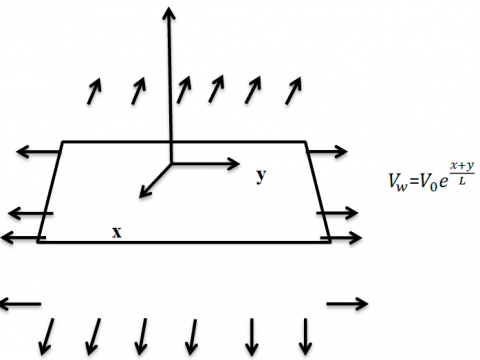Figure 1. Flow geometry

$T_{w}=T_{\infty}+T_{0} e^{\frac{A(x+y)}{2 L}}$

$U_{w}=U_{0} e^{\frac{x+y}{L}}$

By taking above assumptions into account, the laws of conservation of momentum, mass, and energy are given by

$\boldsymbol{\nabla} \cdot \mathbf{V}=0$ (1)

$\rho_{n f} \frac{d \mathbf{V}}{d t}=\operatorname{div} \boldsymbol{T}^{*}$ (2)

$\left(\rho C_{p}\right)_{n f} \frac{d T}{d t}=k_{n f} \nabla^{2} T$ (3)

where the velocity is of the form

$V=[u(x, y, z), v(x, y, z), w(x, y, z)]$ (4)

The constitutive equations for viscous incompressible fluid are assumed by

$\mathrm{T}^{*}=-p \mathbf{I}+\mu_{n f} \mathbf{A}_{1}$ (5)

$\mathbf{A}_{1}=\mathbf{L}+\mathbf{L}^{T}$ (6)

$\mathbf{L}=\operatorname{grad} \mathbf{V}$ (7)

$\operatorname{grad} \mathbf{V}=\left[\begin{array}{lll}{\frac{\partial u}{\partial x}} & {\frac{\partial u}{\partial y}} & {\frac{\partial u}{\partial z}} \\ {\frac{\partial v}{\partial x}} & {\frac{\partial v}{\partial y}} & {\frac{\partial v}{\partial z}} \\ {\frac{\partial w}{\partial x}} & {\frac{\partial w}{\partial y}} & {\frac{\partial w}{\partial z}}\end{array}\right]$ (8)

Here T* represents the Cauchy stress tensor, (ρcp) nanofluid heat capacity, p denote pressure, d/dt scrutinized the material derivate, knf is the nanofluid thermal conductivity, T refers temperature, I identity tensor and A1 the first Rivlin-Erickson tensor which is defined by

$\mathbf{A}_{1}=\left[\begin{array}{ccc}{2 \frac{\partial u}{\partial x}} & {\frac{\partial v}{\partial x}+\frac{\partial u}{\partial y}} & {\frac{\partial w}{\partial x}+\frac{\partial u}{\partial z}} \\ {\frac{\partial v}{\partial x}+\frac{\partial u}{\partial y}} & {2 \frac{\partial v}{\partial y}} & {\frac{\partial w}{\partial y}+\frac{\partial v}{\partial z}} \\ {\frac{\partial w}{\partial x}+\frac{\partial u}{\partial z}} & {\frac{\partial w}{\partial y}+\frac{\partial v}{\partial z}} & {2 \frac{\partial w}{\partial z}}\end{array}\right]$ (9)

Using Eqns. (4) - (9) in Eq. (2) we get the shear and the normal components of stress tensor  These are given by

$\sigma_{x x}=2 \mu_{n f} \frac{\partial u}{\partial x}$ (10)

$T_{x y}=T_{y x}=\mu_{n f}\left(\frac{\partial v}{\partial x}+\frac{\partial u}{\partial y}\right)$ (11)

$\sigma_{y y}=2 \mu_{n f} \frac{\partial v}{\partial y}$ (12)

$T_{x z}=T_{z x}=\mu_{n f}\left(\frac{\partial w}{\partial x}+\frac{\partial u}{\partial z}\right)$ (13)

$T_{y z}=T_{z y}=\mu_{n f}\left(\frac{\partial w}{\partial y}+\frac{\partial v}{\partial z}\right)$ (14)

$\sigma_{z z}=2 \mu_{n f} \frac{\partial w}{\partial z}$ (15)

Invoking above results in Eq. (2) we get x, and z components of the momentum equation. The components are given by

$\rho_{n f} \frac{d u}{d t}=-\frac{\partial p}{\partial x}+2 \mu_{n f} \frac{\partial^{2} u}{\partial x^{2}}+\mu_{n f} \frac{\partial^{2} u}{\partial y^{2}}+\mu_{n f} \frac{\partial^{2} v}{\partial x \partial y}+$

$\mu_{n f} \frac{\partial^{2} u}{\partial z^{2}}+\mu_{n f} \frac{\partial^{2} w}{\partial z \partial x}$ (16)

$\rho_{n f} \frac{d v}{d t}=-\frac{\partial p}{\partial y}+\mu_{n f} \frac{\partial^{2} u}{\partial x \partial y}+\mu_{n f} \frac{\partial^{2} v}{\partial x^{2}}+2 \mu_{n f} \frac{\partial^{2} v}{\partial y^{2}}+$

$\mu_{n f} \frac{\partial^{2} v}{\partial z^{2}}+\mu_{n f} \frac{\partial^{2} w}{\partial z \partial y}$ (17)

$\rho_{n f} \frac{d w}{d t}=-\frac{\partial p}{\partial z}+\mu_{n f} \frac{\partial^{2} w}{\partial x^{2}}+\mu_{n f} \frac{\partial^{2} u}{\partial x \partial z}+\mu_{n f} \frac{\partial^{2} w}{\partial y^{2}}+$

$\mu_{n f} \frac{\partial^{2} v}{\partial y \partial z}+2 \mu_{n f} \frac{\partial^{2} w}{\partial z^{2}}$ (18)

With the help of boundary layer approach and Eq. (1), we find that Eq. (18) vanishes and Eq. (16)-(17) take the form

$u \frac{\partial u}{\partial x}+v \frac{\partial u}{\partial y}+w \frac{\partial u}{\partial z}=-\frac{1}{\rho_{n f}} \frac{\partial p}{\partial x}+v_{n f} \frac{\partial^{2} u}{\partial z^{2}}-\frac{v_{n f} \varepsilon}{K} u$ (19)

$u \frac{\partial v}{\partial x}+v \frac{\partial v}{\partial y}+w \frac{\partial v}{\partial z}=-\frac{1}{\rho_{n f}} \frac{\partial p}{\partial y}+v_{n f} \frac{\partial^{2} v}{\partial z^{2}}-\frac{v_{n f} \varepsilon}{K} v$ (20)

After invoking boundary layer approximation, energy Eq. (3) becomes

$u \frac{\partial T}{\partial x}+v \frac{\partial T}{\partial y}+w \frac{\partial T}{\partial z}=\alpha_{n f} \frac{\partial^{2} T}{\partial z^{2}}$ (21)

Here boundary conditions are

$u=U_{w}, v=V_{w}, w=0, T=T_{w} \quad z=0$ (22)

$u=U_{s}(x, y), v=V_{s}(x, y), w=0, T=T_{\infty} \quad z \rightarrow$ (23)

Here subscript ‘w’ refers conditions at the wall. This consideration accepts that the stretching sheet velocities and the wall temperature are define as

$U_{w}=U_{0} e^{\frac{y+x}{L}}, V_{w}=V_{0} e^{\frac{y+x}{L}}, T_{w}=T_{\infty}+T_{0} e^{\frac{A(y+x)}{2 L}}$ (24)

$U_{s}(x, y)=U_{e} e^{\frac{x+y}{L}}, V_{s}(x, y)=V_{e} e^{\frac{x+y}{L}}$ (25)

$\rho_{n f}$ represents density, $\mu_{n f}$ stand for viscosity, $v_{n f}$ designated the kinematic velocity and $\alpha_{n f}$ represent thermal diffusivity of nanofluid defined as follow.

$\mu_{n f}=\frac{\mu_{f}}{(1-\phi)^{2.5}}, \rho_{n f}=(1-\phi) \rho_{f}+\phi \rho_{s}$ (26)

$\left(\rho C_{p}\right)_{n f}=(1-\phi)\left(\rho c_{p}\right)_{f}+\phi\left(\rho c_{p}\right)_{s^{\prime}} v_{n f}=\frac{\mu_{n f}}{\rho_{n f}}$ (27)

$\frac{k_{n f}}{k_{f}}=\frac{\left(k_{S}+2 k_{f}\right)-2 \phi\left(k_{f}-k_{S}\right)}{\left(k_{S}+2 k_{f}\right)+\phi\left(k_{f}-k_{S}\right)}, \alpha_{n f}=\frac{k_{n f}}{\left(\rho C_{p}\right)_{n f}}$ (28)

Using Eq. (23) in Eqns. (19)-(20) we get

$-\frac{1}{\rho_{n f}} \frac{\partial p}{\partial x}=\frac{1}{L}\left(U_{e}^{2}+U_{e} V_{e}\right) e^{\frac{2(x+y)}{L}}$, $-\frac{1}{\rho_{n f}} \frac{\partial p}{\partial y}=\left(U_{e} V_{e}+V_{e}^{2}\right) \frac{1}{L} e^{\frac{2(x+y)}{L}}$ (29)

Thus Eq. (19) and (20) become

$\frac{\partial u}{\partial x}+v \frac{\partial u}{\partial y}+w \frac{\partial u}{\partial z}=\frac{1}{L}\left(U_{e}^{2}+U_{e} V_{e}\right) e^{\frac{2(x+y)}{L}}+v_{n f} \frac{\partial^{2} u}{\partial z^{2}}$ (30)

$u \frac{\partial v}{\partial x}+v \frac{\partial v}{\partial y}+w \frac{\partial v}{\partial z}=\frac{1}{L}\left(U_{e} V_{e}+V_{e}^{2}\right) e^{\frac{2(x+y)}{L}}+v_{n f} \frac{\partial^{2} v}{\partial z^{2}}$ (31)

provoking the similarity transformation

$u=U_{e} e^{\frac{x+y}{L}} f^{\prime}(\eta), v=U_{e} e^{\frac{x+y}{L}} g^{\prime}(\eta)$

$w=-\left(\frac{v U_{e}}{2 L}\right)^{1 / 2} e^{\frac{(x+y)}{2 L}}\left[f+\eta f^{\prime}+g+\eta g^{\prime}\right]$

$T=T_{\infty}+T_{0} e^{\frac{A(x+y)}{2 L}} \theta(\eta), \eta=\left(\frac{U_{e}}{2 v L}\right)^{1 / 2} e^{\frac{(x+y)}{2 L} z}$ (32)

and Equation (30)-(31) are transformed to the boundary value problem depicted in the equations below:

$\frac{(1-\phi)^{-2.5}}{\left(1-\phi+\phi\left(\rho_{s} / \rho_{f}\right)\right)} f^{\prime \prime}=$

$\quad-(g+f) f^{\prime \prime}+2\left(g^{\prime}+f^{\prime}\right) f^{\prime}-2\left(1+r_{1}\right)-\delta f^{\prime}$ (33)

$\frac{(1-\phi)^{-2.5}}{\left(1-\phi+\phi\left(\rho_{s} / \rho_{f}\right)\right)} g^{\prime \prime \prime}=$

$-(g+f) g^{\prime \prime}+2\left(g^{\prime}+f^{\prime}\right) g^{\prime}-2 r_{1}\left(1+r_{1}\right)$ (34)

$\left[\frac{k_{n f} / k_{f}}{1-\phi+\phi\left(\left(\rho c_{p}\right)_{s} /\left(\rho c_{p}\right)_{f}\right)}\right] \frac{1}{P r} \theta^{*}$$-(g+f) \theta^{\prime}+A\left(g^{\prime}+f^{\prime}\right) \theta$ (35)

And equivalent boundary conditions (22) and (23) become

$g(0)=f(0)=0, f^{\prime}(0)=\alpha_{1}, g^{\prime}(0)=\alpha_{2}, \theta(0)=1$ (36)

$f^{\prime}(\infty)=1, g^{\prime}(\infty)=r_{1}, \theta(\infty)=0$ (37)

The parameters of physical characteristics are the dimensionless surface friction drag coefficients Cfx and Cfy and the heat transfer rate or Nusselt number demarcated as

\begin{aligned} c_{f x} &=\frac{\tau_{w x}}{\rho_{f} U_{0}^{2} / 2} \\ c_{f y}=& \frac{\tau_{w y}}{\rho_{f} U_{0}^{2} / 2} \\ N u_{x} &=\frac{x q_{w}}{k_{f}\left(T-T_{\infty}\right)} \end{aligned} (38)

The respective shear stresses are signified with and in the x-, y- directions, respectively, and qw signifies surface heat flux

$\tau_{w x}=\mu_{n f}\left(\frac{\partial w}{\partial x}+\frac{\partial u}{\partial z}\right)_{z=0}$

$\tau_{w y}=\mu_{n f}\left(\frac{\partial w}{\partial y}+\frac{\partial v}{\partial z}\right)_{z=0}$

$q_{w}=-k_{n f}\left.\frac{\partial T}{\partial z}\right|_{z=0}$ (39)

Using Eq. (32) and (39), we get

$R e_{x}^{1 / 2} C_{f x} / 2 e^{\frac{3(x+y)}{2 L}}=\frac{1}{(1-\phi)^{2.5}} f^{\prime \prime}(0)$ (40)

$R e_{y}^{1 / 2} C_{f j} / 2 e^{\frac{3(x+y)}{2 L}}=\frac{1}{(1-\phi)^{2.5}} g^{\prime \prime}(0)$ (41)

$R e_{x}^{-1 / 2} N_{u_{x}} / 2 e^{\frac{x+y}{2 L}} \frac{x}{L}=-\frac{k_{n f}}{k_{f}} \theta^{\prime}(0)$ (42)

where Re denote the Reynolds number given as $R e=U_{0} L / v$.

3. Optimal Homotopy Analysis Method

To obtain solutions of Eqns. (33-35), we utilized optimal homotopy analysis method (Optimal HAM). This powerful tool is used for the consequences of boundary value problem. The initial guess and corresponding operators for the method are defined as

$f_{0}(\eta)=\eta+\left(\alpha_{1}-1\right) *(1-\exp (-\eta))$ (43)

$g_{0}(\eta)=\left(\alpha_{2}-r_{1}\right)+r_{1} * \eta+\left(r_{1}-\alpha_{2}\right) *(1-\exp (-\eta))$ (44)

$\theta_{0}(\eta)=\exp (-\eta * P r)$ (45)

$\mathcal{L}_{f}=\frac{d^{3} f}{d \eta^{3}}-\frac{d f}{d \eta}$ (46)

$\mathcal{L}_{f}\left[C_{1}+C_{2} \exp (\eta)+C_{3} \exp (-\eta)\right]=0$ (47)

$\mathcal{L}_{g}=\frac{d^{3} g}{d \eta^{3}}-\frac{d g}{d \eta}$ (48)

$\mathcal{L}_{g}\left[C_{4}+C_{5} \exp (\eta)+C_{6} \exp (-\eta)\right]=0$ (49)

$\mathcal{L}_{\theta}=\frac{d^{2} \theta}{d \eta^{2}}+\operatorname{Pr} \frac{d \theta}{d \eta}$ (50)

$\mathcal{L}_{\theta}\left[C_{7}+C_{8} \exp \left(-\eta^{*} \boldsymbol{P} \boldsymbol{r}\right)\right]=0$ (51)

where $c_{i}(i=1,2, \ldots 8)$ are arbitrary constants. If $q \in[0,1]$ denotes embedding parameter. Here $\hbar_{f}, \hbar_{g}$ and are subsidiary parameters, not equal to zero. In order to write zeroth and mth order deformation problems we proceed as follows:

4. Convergence of Oham Solution

Optimal HAM gives more flexibility to control the convergence region for the series solution. Specific values are assigned to the auxiliary parameters ℏf, ℏg and ℏө for obtaining the convergence solutions. The residual errors for the boundary value problem are examined. These residual errors are listed in the Tables 1 and 2 below. The obtained convergence in the tables claim that the values given to the auxiliary parameters and remaining appeared parameters in the flow are good to use.

Table 1. Average residual square error ∈mt

 $\frac{\text { values } \rightarrow}{\text { order } \downarrow}$ $\hbar_{f}$ $\hbar_{g}$ $\hbar_{\theta}$ $\epsilon_{m}^{t}$ 2 -0.32861 -0.64120 -1.00311 $1.92312 \times 10^{-5}$ 4 -0.38912 -0.69310 -1.043101 $7.03031 \times 10^{-6}$ 6 -0.40021 -0.74001 -1.100430 $1.032168 \times 10^{-6}$ 8 -0.44091 -0.80001 -1.32109 $8.92138 \times 10^{-7}$ 10 -0.65178 -0.83108 -1.67091 $0.71201 \times 10^{-9}$

Table 2. Individual residual square errors for $\epsilon_{m}^{f}, \epsilon_{m}^{g},$ and $\in_{m}^{\theta}$

 $\frac{\text { values } \rightarrow}{\text { order } \downarrow}$ $\frac{\hbar_{f}=-0.65178}{\epsilon_{m}^{f}}$ $\frac{\hbar_{g}=-0.83108}{\epsilon_{m}^{g}}$ $\frac{\hbar_{\theta}=-1.67091}{\epsilon_{m}^{\theta}}$ 10 $3.81201 \times 10^{-7}$ $3.61209 \times 10^{-6}$ $3.61293 \times 10^{-6}$ 12 $1.91034 \times 10^{-10}$ $9.62340 \times 10^{-9}$ $1.54128 \times 10^{-6}$ 16 $5.31920 \times 10^{-11}$ $9.62340 \times 10^{-9}$ $7.42987 \times 10^{-7}$ 18 $8.75420 \times 10^{-13}$ $1.00412 \times 10^{-13}$ $3.39086 \times 10^{-9}$ 20 $1.52139 \times 10^{-19}$ $6.92345 \times 10^{-17}$ $1.42980 \times 10^{-9}$

4.1 Result and discussion

This section is developed to characterize and scrutinize the results examined for the present flow analysis. Impacts of sundry dimensionless parameters on the friction drag, axial velocity, heat transfer rate, and temperature field are exhibited. The suggested parameters include the solid volume fraction $\phi$, r1 the ratio or stagnation parameter, α1 and α2 stretching parameter, and A the exponent temperature parameter for water based nanofluid are dig out in Figures 2-10. Three distinct nanoparticles Cu (Copper), Al2O3 (Alumina), and TiO2 (Titania) are scrutinized in the present flow. Keep in mind that these particles were also examined by the Oztop and Abu-Nada . During the scrutiny of by Oztop and Abu-Nada , the parameters were taken $\phi$ is taken from 0 to 0.2 (0≤$\phi$≤0.2), here $\phi$=0, depicts the case of flow where the nanoparticles are absent and only base fluid remains, and for water based nanofluid Prandtl number Pr as 6.2. The thermophysical characteristics of the base-fluid and distinct nanoparticles, namely, Cu (Copper), Al2O3 (Alumina), and TiO2 (Titania) are tabulated in Table 2. This section contains Figures 2-10. The results are exhibited in order to dig out the impacts of sundry parameters on temperature and velocity performance. Table 1 is prepared to access the number of approximation order, required for convergent solution. Here we found that 30th order of approximation is sufficient. Figures 2-5 examines the impacts of Cu (Copper) and r1 the ratio or stagnation parameter on the axial velocity and temperature field. We depicted that enhancing the solid volume friction $\phi$ of Cu (Copper) results in the enhancement of temperature field and velocity field. The velocity field arises, whereas, the temperature field declines for the ratio parameter r1(≥α2).  Figures 8-10 elucidate the influence of distinct nanoparticles i.e., Cu (Copper), Al2O3 (Alumina), and TiO2 (Titania) on the flow under consideration. Figures 6 and 7 depicts that for Al2O3 (Alumina)-water nanofluids leads to thick the momentum boundary as compare to Cu (Copper) and TiO2 (Titania). The thicker boundary layer for temperature field is dig out for the nanoparticle TiO2 (Titania) and base fluid water (H20) shown in Figure 8 as compare to Cu (Copper) and Al2O3 (Alumina) nanoparticles. Thicker thermal boundary layer is dig out for the arising exponent temperature parameter A. Impacts of arisen parameters on the friction drag along x,y−direction is delineated in Figure 9. The friction drag enhances along x,y−direction for large values of ratio parameter, whereas, it declines for stretching parameters α1 and α2.

## 2.pngFigure 2. Error decay approximation of order 8

## 3.pngFigure 3. Influence of Cu-water based and r1 on f'(η)

## 4.png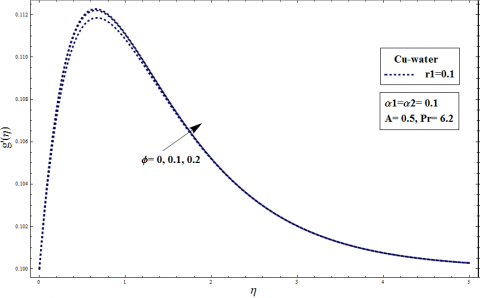Figure 4. Effect of Cu-water based on g'(η) at r 1=1.05

## 5.pngFigure 5. Influence of Cu-nanoparticle volume fractions on g′(η) at r1=0.5

## 6.png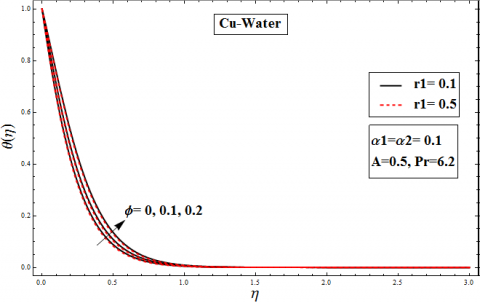Figure 6. Impact of Cu-nanoparticle volume fractions and r1 on (η)

## 7.pngFigure 7. Impact of nanoparticle on f'(η)

## 8.png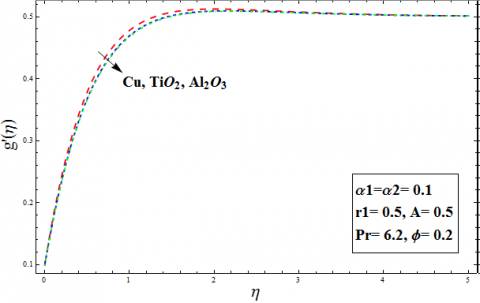Figure 8. Effect of nanoparticle on g′(η)

## 9.pngFigure 9. Inspiration of nanoparticles and temperature exponent A on θ(η)

## 10.pngFigure 10. Impact of $\phi$ for distinct nanoparticles on friction drag along x−direction when r1=0,5,1

## 11.png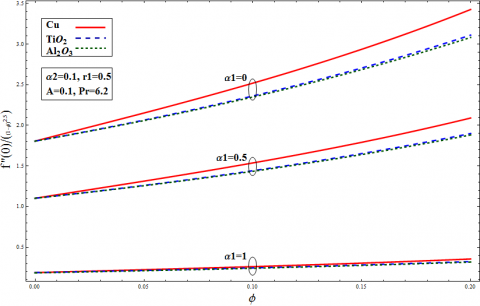Figure 11. Impact of $\phi$ for distinct nanoparticles on Skin-friction coefficient along x−direction when α1=0,0.5

4.2 Conclusions

The effects of stagnation point on the two-phase flow of nanofluid past an exponentially stretching sheet are discovered. The arising nonlinear problem is computed. The present study reveals following key points.

The solid volume fraction $\phi$ appreciably increases the dimensionless axial velocity and temperature field. It also increases heat flux and skin friction.

Table 3. Thermophysical characteristics of water and nanoparticles

 Physical Properties Base-Fluid (water) $\mathbf{C} \mathbf{u}$ $\mathbf{A l}_{2} \mathbf{O}_{3}$ $\mathrm{TiO}_{2}$ $\boldsymbol{C}_{\boldsymbol{p}}(\mathbf{J} / \mathbf{k} \mathbf{g} \mathbf{K})$ 4179 385 765 686.2 $\boldsymbol{\rho}\left(\mathbf{k} \mathbf{g} / \mathbf{m}^{3}\right)$ 997.1 8933 3970 4250 $\boldsymbol{k}(\mathbf{W} / \mathbf{m} \mathbf{K})$ 0.613 400 40 8.9538

Table 4. Numerical simulations for friction drag and Nusselt number when $\alpha \mathbf{1}=\alpha 2=0.1$ and $P r=6.2 . \phi$
 $\phi \downarrow$ A r1 $f^{\prime \prime}(\mathbf{0}) /(\mathbf{1}-\boldsymbol{\phi})^{25 / 10}$ $\boldsymbol{g}^{\prime \prime}(\mathbf{0}) /(\mathbf{1}-\boldsymbol{\phi})^{25 / 10}$ $-\boldsymbol{k}_{\boldsymbol{n} f} / \boldsymbol{k}_{f} \boldsymbol{\theta}^{\prime}(\mathbf{0})$ 0.1 -0.5 0.5 -2.91470 (Cu) -1.35140 (Cu) 1.29210 (Cu) -2.5048( $\mathrm{TiO}_{2}$ ) -1.1613( $\mathrm{TiO}_{2}$ ) 1.2437( $\mathrm{Al}_{2} \mathrm{O}_{3}$ ) -2.4781( $\mathrm{Al}_{2} \mathrm{O}_{3}$ ) -1.4190( $\mathrm{Al}_{2} \mathrm{O}_{3}$ ) 1.2170( $\mathrm{TiO}_{2}$ ) 1.5 -3.78720(Cu) -5.81340(Cu) 1.61950(Cu) -3.2545( $\mathrm{TiO}_{2}$ ) -4.9958( $\mathrm{TiO}_{2}$ ) 1.5519( $\mathrm{Al}_{2} \mathrm{O}_{3}$ ) -3.2199( $\mathrm{Al}_{2} \mathrm{O}_{3}$ ) -4.9426( $\mathrm{Al}_{2} \mathrm{O}_{3}$ ) 1.5176( $\mathrm{TiO}_{2}$ ) 0.2 0.5 0.5 -4.05680(Cu) -1.88080(Cu) 2.92980(Cu) -3.2395( $\mathrm{TiO}_{2}$ ) -1.5019( $\mathrm{TiO}_{2}$ ) 2.7360( $\mathrm{Al}_{2} \mathrm{O}_{3}$ ) -3.1839( $\mathrm{Al}_{2} \mathrm{O}_{3}$ ) -1.4762( $\mathrm{Al}_{2} \mathrm{O}_{3}$ ) 2.6213( $\mathrm{TiO}_{2}$ ) 1.5 -5.27130(Cu) -8.09140(Cu) 3.66880(Cu) -4.20900( $\mathrm{TiO}_{2}$ ) -6.4610( $\mathrm{TiO}_{2}$ ) 3.4109( $\mathrm{Al}_{2} \mathrm{O}_{3}$ ) -4.1369( $\mathrm{Al}_{2} \mathrm{O}_{3}$ ) -6.3501( $\mathrm{Al}_{2} \mathrm{O}_{3}$ ) 3.2669( $\mathrm{TiO}_{2}$ )

The ratio/stagnation parameter r1 enhances the velocity and temperature profile.

The impacts of temperature exponent A increases dimensionless temperature, heat flux, and thermal boundary layer.

The friction drag along x,−direction enhances for Cu (Copper)- H20(water) nanofluid and decline for Al2O3 (Alumina)- H20(water) nanofluid shown in Table 4.

The Nusselt number along x,−direction enhances for Cu (Copper)- H20(water) nanofluid and decline for TiO2 (Titania)- H20(water) nanofluid shown in Table 4.

Acknowledgement

The authors are greatly obliged and thankful to the reviewers for their valued suggestions which helped to improve the manuscript. The authors are thankful to the University of Management and Technology Lahore, Pakistan for facilitating and supporting the research work.

References

 Sakiadis, B.C. (1961). Boundary- layer behavior on continuous solid surface I. Boundary layer equations for two dimensional and axisymmetric flow. Journal American Institute of Chemical Engineers, 7: 26-28. https://doi.org/10.1002/aic.690070108

 Sakiadis, B.C. (1961). Boundary layer behavior on continuous solid surface. II. Boundary layer on a continuous flat surface. Journal American Institute of Chemical Engineers, 7: 221-225. https://doi.org/10.1002/aic.690070211

 Crane, L.J. (1985). Flow past a stretching plate. Zeitschrift fur Angewandte Mathematik and Physik, 21(4): 645-647.

 Grubka, L.J., Bobba, K.M. (1985). Heat transfer characteristics of a continuous stretching surface with variable temperature. J. Heat Transfer, 107: 248-250. https://doi.org/10.1115/1.3247387

 Ali, M.E. (1995). On thermal boundary layer on a power-law stretched surface with suction and injection. Int. J. Heat Fluid Flow, 16: 280-290.

 Andersson, H.I. (1992). MHD flow of a viscoelastic fluid past a stretching surface. Acta Mechanica, 95: 227-230.

 Prasad, K.V., Abel, S., Datti, P.S. (2003). Diffusion of chemically reactive species of a non-Newtonian fluid immersed in a porous medium over a stretching sheet. International Journal of Non-Linear Mechanics, 38(5): 651-657. https://doi.org/10.1016/S0020-7462(01)00122-6

 Liu, I.C. (2005). Flow and heat transfer of an electrically conducting fluid of second grade in porous medium over a stretching sheet subject to a transverse magnetic field. International Journal of Non-Linear Mechanics, 40(4): 465-474. https://doi.org/10.1016/j.ijnonlinmec.2004.07.008

 Chen, C.H. (2010). On the analytic solution of MHD flow and heat transfer for two types of viscoelastic fluid over a stretching sheet with energy dissipation, internal heat source and thermal radiation. International Journal of Heat and Mass Transfer, 53(19-20): 4264-4273. https://doi.org/10.1016/j.ijheatmasstransfer.2010.05.053

 Magyari, E., Keller, B. (1999). Heat and mass transfer in the boundary layer on an exponentially stretching continuous surface. Journal of Physics D: Applied Physics, 32(5): 577-585.

 Elbashbeshy, E.M.A. (2001). Heat transfer over an exponentially stretching continuous surface with suction. Archives of Mechanics, 53(6): 643-651.

 Khan, S.K., Sanjayanand, E. (2005). Viscoelastic boundary layer flow and heat transfer over an exponentially stretching sheet. International Journal of Heat and Mass Transfer, 48: 1534-1542. https://doi.org/10.1016/j.ijheatmasstransfer.2004.10.032

 Khan, S.K. (2006). Boundary layer viscoelastic fluid flow over an exponentially stretching sheet. International Journal of Applied Mechanics and Engineering, 11(2): 321-335.

 Partha, M.K., Murthy, P.V.S.N., Rajasekhar, G.P. (2005). Effect of viscous dissipation on the mixed convection heat transfer from an exponentially stretching surface. Heat Mass Transfer, 41(4): 360-366. https://doi.org/10.1007/s00231-004-0552-2

 Abd El-Aziz, M. (2009). Viscous dissipation effect on mixed convection flow of a micropolar fluid over an exponentially stretching sheet. Canadian Journal of Physics, 87(4): 359-368. https://doi.org/10.1139/P09-047

 Pal, D. (2010). Mixed convection heat transfer in the boundary layer on an exponentially stretching surface with magnetic field. Applied Mathematics and Computation, 217(6): 2356-2369. https://doi.org/10.1016/j.amc.2010.07.035

 Nadeem, S., Zaheer, S., Fang, T. (2011). Effects of thermal radiation on the boundary layer flow of a Jeffrey fluid over an exponentially stretching surface. Numerical Algorithms, 57: 187-205. https://doi.org/10.1007/s11075-010-9423-8

 Bhattacharyya, K., Vajravelu, K. (2012). Stagnation-point flow and heat transfer over ab exponentially shrinking sheet. Communications in Nonlinear Science and Numerical Simulation, 17(7): 2728-2734. https://doi.org/10.1016/j.cnsns.2011.11.011

 Nadeem, S., Lee, C.H. (2012). Boundary layer flow of nanofluid over an exponentially stretching surface. Nanoscale Research Letters, 7(1): 94. https://doi.org/10.1186/1556-276X-7-94

 Liu, C., Wang H.H., Peng, Y.F. (2013). Flow and heat transfer for three-dimensional flow over an exponentially stretching surface. Chemical Engineering Communications, 200(2): 253-268. https://doi.org/10.1080/00986445.2012.703148

 Khan, W., Pop, I. (2010). Boundary-layer flow of a nanofluid past a stretching sheet. International Journal of Heat and Mass Transfer, 53: 2477-2483. https://doi.org/10.1016/j.ijheatmasstransfer.2010.01.032

 Nadeem, S., Haq, R.U., Khan, Z.H. (2013). Numerical solution of non-Newtonian nanofluid flow over a stretching sheet. Applied Nanosciences, 4(5): 625-631. https://doi.org/10.1007/s13204-013-0235-8

 Nadeem, S., Haq, R.U., Khan, Z.H. (2014). Heat transfer analysis of water-based nanofluid over an exponentially stretching sheet. Alexandria Engineering Journal, 53(1): 219-224. https://doi.org/10.1016/j.aej.2013.11.003